Home > ACC7 > Chapter cc39 > Lesson cc39.2.5 > Problem9-132

9-132.
1. Simplify each of the following expressions. Homework Help ✎

1.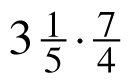2.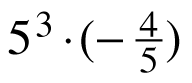3.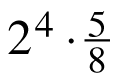4.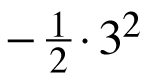5.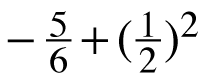6.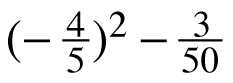7.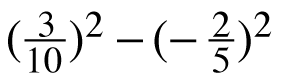8.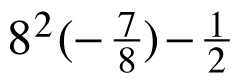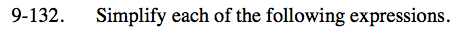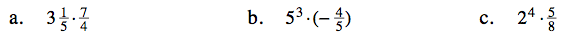Convert the mixed number into a fraction greater than one.

$\frac{16}{5}\left(\frac{7}{4}\right)$

$\frac{28}{5} = 5\frac{3}{5}$

$\frac{4}{5} = (-1)(4)\left(\frac{1}{5}\right)$

Notice that the 5 in the denominator would have an exponent of –1.

5(3 − 1)(−4)

52(−4) = −100

This can be rewritten as:

$2^{4}\left(\frac{5}{2^{3}}\right)$

See part (b).

10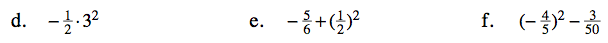Use the Order of Operations.

Use the Order of Operations.

$-\frac{5}{6} + \frac{1}{4}$

$-\frac{20}{24} + \frac{6}{24} = -\frac{14}{24}$

Notice that squaring a negative number has the same result as multiplying two negative numbers: the product is always positive.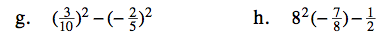Follow the Order of Operations.

$8^{(2-1)}(-7)-\frac{1}{2}$

$8(-7) - \frac{1}{2}$

$(-56) - \frac{1}{2}$

$-56\frac{1}{2}$